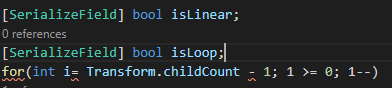# Customizable PatrolPath

Hello everyone!

Here goes, I want to make it so that in the inspector, I could decide if the Path is either linear (enemies will go from start to finish, then from finish to start etc), or loop (the way we have done).

All I came up with is this, which I found on the web.I did try to make changes to the GetNexIndex but it ends up in a big mess. Any suggestion?

There are actually only two values in your plan… the path is linear, or the path is a loop. We can simply the declaration to simply

``````[SerializeField] bool isLinear; //if it isn't linear, it's a loop
``````

The tricky part is differentiating between behavior when it’s a loop or when it’s linear. We want the PatrolPath to be stateless, you might have several guards following the same path. At the AIController level, we’ll need to ask PatrolPath if it’s Linear or a Loop, and call the appropriate method…

``````     public bool IsLinear()
{
return isLinear;
}

public int GetNextIndex(int i)
{
if (i + 1 == transform.childCount)
{
return 0;
}
return i + 1;
}

public int GetNextIndex(int i, ref int direction)
{
int result = i + direction;
if (result >= transform.childCount || result <0)
{
direction *= -1;
return i + direction;
}
return result;
}
``````

In AIController, for CycleWaypoint, we’ll need a slight tweak to our code:

``````        private int direction = 1;

private void CycleWaypoint()
{
if (patrolPath.IsLinear())
{
currentWaypointIndex = patrolPath.GetNextIndex(currentWaypointIndex, ref direction);
}
else
{
currentWaypointIndex = patrolPath.GetNextIndex(currentWaypointIndex);
}
}
``````
1 Like

Hello Brian!

Thank you for the response ! I didn’t even think of inserting a direction! I will give it a try right away and see how it goes

Hello again!

Thank you Brian! I tried and… it kinda worked:

First, the Gizmos is great

It was working fine but as I anticipated, the enemy goes back and forth between the last waypoint and the one before!I tried to alter the direction with thisBut it created this

Well I will try and look more into it! Le you know if I come up with something

Let’s go back to the GetNextIndex I made for my example:

``````        public int GetNextLinearIndex(int i, ref int direction)
{
int result = i + direction;
if (result >= transform.childCount || result <0)
{
direction *= -1;
return i + direction;
}
return result;
}
``````

Here’s how it works:
We start by calculating what our next waypoint should be based on the direction passed into the method. Direction should only ever be 1 or -1, assuming it is never altered outside of this formula and it’s initialized correctly as a global variable in your AI Controller.

So Let’s assume we have 5 waypoints. In our AIController, the first waypoint is zero. We’ve arrived there, and want the next waypoint, so we CycleWaypoint

``````        private void CycleWaypoint()
{
if (patrolPath.IsLinear())
{
currentWaypointIndex = patrolPath.GetNextLinearIndex(currentWaypointIndex, ref direction);
}
else
{
currentWaypointIndex = patrolPath.GetNextIndex(currentWaypointIndex);
}
}
``````

So GetNextLinearIndex() should be getting a currentIndex of 0, and a direction of 1. It adds the two together, 0+1=1

Then it does it’s bounds check to make sure that the index isn’t greater than the number or children or less than 0. Our result is 1, so we don’t touch direction, and we return 1.

This continues until our currentIndex is 4. When it’s 4, the GetLinearIndex is sent (4,1). Our method gets a result of 5, which is equal to the child count. We can’t return 5 because our index is zero based, it’s time to go the other way. So the direction is flipped (1 * -1 = 1), and we send a new result of i+direction which is now 4 + -1 = 3

Now direction has become -1, and we continue down our path. The next call to CycleWaypoint() will be sending (3,-1) with a result of 2 and the ref remaining unchanged at -1
(2, -1) = 1
(1, -1) = 0
(0, -1) We’re now in the special case where the result (-1) is out of bounds. Once again, we flip the direction (-1 * -1 = 1) and return the passed index + direction (0 + 1 = 1).

So here’s the whole result cycle

``````(0,1) = 1
(1,1) = 2
(2,1) = 3
(3,1) = 4
(4,1) = 3 with the direction changed to -1
(3, -1) = 2
(2, -1) = 1
(1, -1) = 0
(0, -1) = 1 with the direction changed to 1
[cycle repeats]
``````

For your OnDrawGizmos(), it doesn’t matter that the direction is within the for block, because it’s still going to flip only once anyways, and it will still draw correctly.

I anticipate that this is because in AIController, you’re declaring int direction = 1; inside the CycleWaypoint() method instead of outside the method. direction should be a global variable. If it’s declared within CycleWaypoint() then you get

``````(0,1) = 1
(1,1) = 2
(2,1) = 3
(3,1) = 4
(4,1) = 3 direction is changed to -1, but is discarded because direction is a local
(3,1) = 4
(4,1) = 3...this will continue between the last and next to last element
``````
2 Likes

This topic was automatically closed 20 days after the last reply. New replies are no longer allowed.